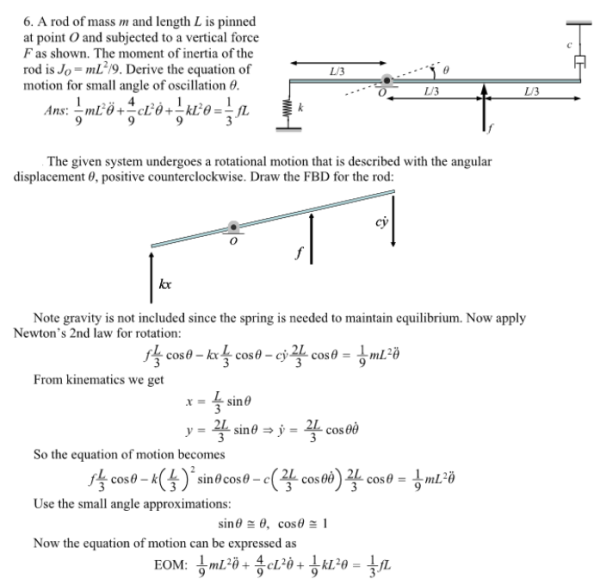A rod of mass m and length L is pinned at point O and subjected to a vertical force F as shown. The moment of inertia of the rod is Jo=mL^2/9. Derive the equation of motion for small angle of oscillation theta.A rod of mass m and length L is pinned at point O and subjected to a vertical force F as shown. The moment of inertia of the rod is Jo=mL^2/9. Derive the equation of motion for small angle of oscillation theta.

System Dynamics Page 2 dynamics dynamics dynamics dynamics dynamics dynamics dynamics System dynamics Page 3